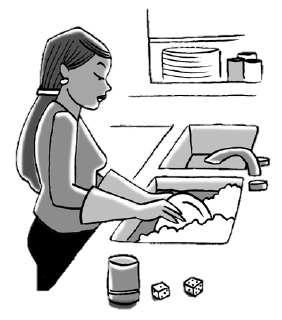### Home > INT3 > Chapter 6 > Lesson 6.1.2 > Problem6-16

6-16.

Myriah hates doing the dishes, but her parents insist that she must help out and take a turn sometimes. Myriah is only willing to do the dishes sometimes, so her mom proposes that they roll two dice. If the sum of the dice is $6$ or less, Myriah will do the dishes. If the sum is $7$ or more, one of her parents will do the dishes.

How many days each week can Myriah expect to have to do the dishes? Create a simulation of rolling the two dice (generate two random integers between $1$ and $6$) and record the sum. Run the simulation $30$ times to determine how often Myriah can expect to do the dishes.

Use a random number generator to generate $60$ random integers from $1$ to $6$.

Record the sum of each pair of numbers ($60$ numbers is $30$ pairs).

How many pairs of numbers have a sum of $7$ or more?